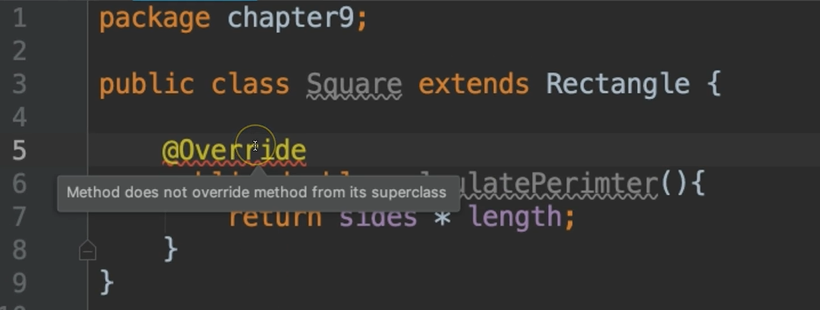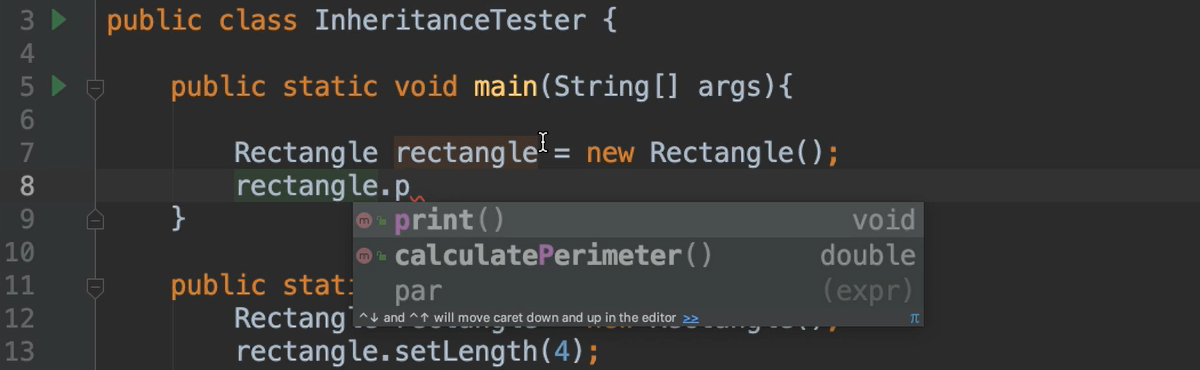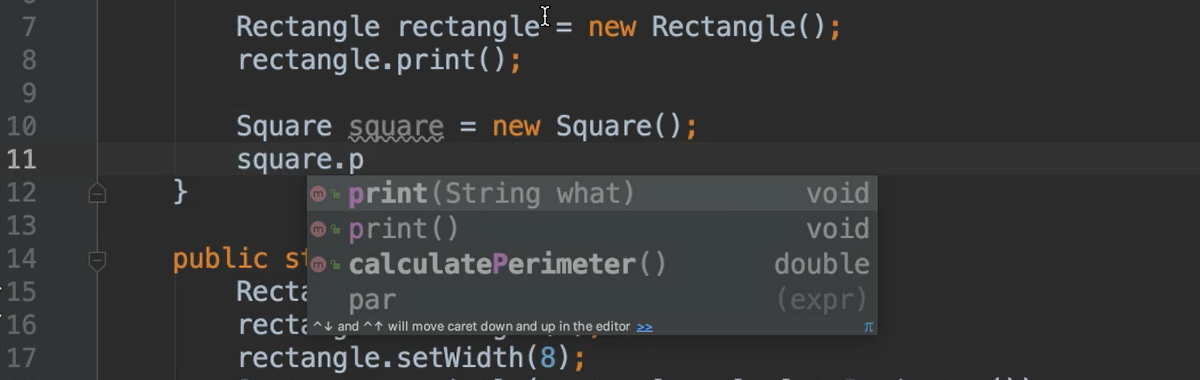Transcripted Summary

Again, a subclass inherits the members of its parent, however, a subclass may want to change the functionality of a method that it inherited.

This is allowed by overriding the inherited method.

### # Overriding Inherited Methods

For example, a square is a rectangle, meaning we could have a `Square` class that inherits from a `Rectangle` class. And let's say one of the methods that was inherited was to calculate the perimeter.

Well, the formula for calculating the perimeter of a square is different than the formula for calculating the perimeter of a rectangle.

So, while it's great that the `Square` class inherits this method, that class needs to change the behavior of that method. This is called overriding a method.

Let's code this very example.

In this `Rectangle` class, I'm going to create fields for the length, width, and the sides.

In addition to the getters and setters, let's create another method to `calculatePerimeter()`.

### # Rectangle.java

``````package chapter9;

public class Rectangle {

protected double length;
protected double width;
protected double sides = 4;

public double getLength() {
return length;
}

public void setLength(double length) {
this.length = length;
}

public double getWidth() {
return width;
}

public void setWidth(double width) {
this.width = width;
}

public double getSides() {
return sides;
}

public void setSides(double sides) {
this.sides = sides;
}

public double calculatePerimeter(){
return (2 * length) + (2 * width);
}
}
``````

Now we'll have a class called `Square` inherit from `Rectangle`.

We create the inherit relationship (using `extends`) and we get all of the goodies for free but we want to override this `calculatePerimeter()` method in the `Rectangle` class.

To do so, we recreate that method with the same exact signature as the one that exists in `Rectangle`. So, I'm going to just copy this and paste it over here in `Square`, and then we're going to change the implementation of the method.

### # Square Example Code Using Override of Rectangle Class

``````package chapter9;

public class Square extends Rectangle {

public double calculatePerimeter(){
return sides * length;
}
}
``````

The formula to calculate the perimeter of a square is sides times length. And notice, I didn't declare sides or length anywhere in the `Square` class, but I inherited them from the `Rectangle` class.

So, this is how you override a method — use the exact same signature but change the body.

Override Annotation

It's encouraged that you use the override annotation, which is the @ symbol followed by the word Override — `@Override`. This is not required, but it's strongly encouraged. This lets Java know that your intention is to override the method that you inherited from your super class.

Without this override annotation, we could mistakenly think that we're overriding a method, but not really do so.

For example, if I were to comment out the annotation — `//@Override` — and let’s say I were to mistype the name of this method that I’m overriding, then everything would compile, and I would think that I was overriding this method that exists in my super class. However, I goofed up and I mistyped it. So, I wasn’t really overriding anything.

If I add the `@Override` annotation, this will let me know, "No, silly, you haven't overridden anything. This method doesn't exist."So that's a good clue to let us know that we're doing the right thing.

Let me give you a quick refresher. Overloading methods is when you have multiple methods with the exact same name but with different parameter lists.

In Chapter 6, we looked at overloading methods within the same class. When dealing with a subclass, we can overload a method that we have inherited from a superclass, even though that method lives in another class.

Let's update the `Rectangle` and `Square` classes to demonstrate how this is done.

I'm going to create a new method in the `Rectangle` class that simply prints out a statement.

``````public void print(){
System.out.println("I am a rectangle");
}
``````

And then in the `Square` class, I'm going to overload this by creating a method with the same name, but it's going to take a String.

``````public void print(String what){
System.out.println("I am a " + what);
}
``````

Now let's look at this in action.

Notice, when I attempt to call the print method, there's only 1 method available in this `Rectangle` class called `print`.However, when I attempt to call `print` on the `Square` class, there's two.There's the one that we inherited, and also the overloaded one.

We'll run this.

### # InheritanceTester.java

``````package chapter9;

public class InheritanceTester {

public static void main(String[] args){

Rectangle rectangle = new Rectangle();
rectangle.print();

Square square = new Square();
square.print("square");
}
}
``````

And voila!

``````I am a rectangle
I am a square
``````